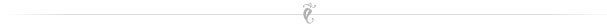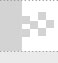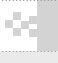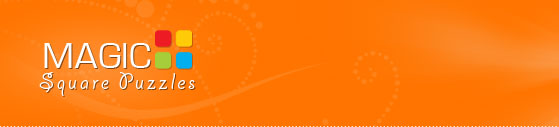Welcome, Guest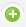Register |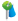Forgot Password |
 Richard Preston “If equations are trains threading the landscape of numbers, then no train stops at pi. ”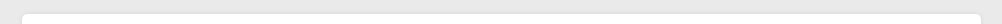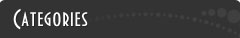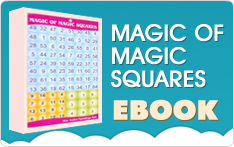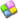The magicsquare game 118# magic square puzzle

••PUZZLE51Solution51Fill the empty cells using numbers from 1 to 64 each only once such that

1) Each row, column and two main diagonals of each 4x4 sub square gives the magic sum of 130.

2) Each 2 x 2 square marked in the same colour gives the sum of 130.

3) The center 2 x 2 marked in black border in each 4x4 sub square gives a sum of 130.

4) Group of cells in boxes within dotted lines is a cage.

Cage sum is mentioned at the top in respective colours.